# Analysis of Stochastic Ensemble Data in SimBiology®

This example shows how to make ensemble runs and how to analyze the generated data in SimBiology®.

### Introduction

When the behavior of a model is stochastic in nature, a single simulation run does not provide enough insight into the model. One has to perform an ensemble of runs. Ensemble runs produce large amounts of data that require systematic analysis.

This example illustrates how to make ensemble runs using SimBiology and how to analyze the generated data.

We will use the G Protein model that was built using published data from Yi et al. (2003). Load the wild-type G Protein model and look at its species and reactions.

```sbioloadproject gprotein_norules m1 m1.Species```
```ans = SimBiology Species Array Index: Compartment: Name: Value: Units: 1 unnamed G 7000 2 unnamed Gd 3000 3 unnamed Ga 0 4 unnamed RL 0 5 unnamed L 6.022e+17 6 unnamed R 10000 7 unnamed Gbg 3000 ```
`m1.Reactions`
```ans = SimBiology Reaction Array Index: Reaction: 1 L + R <-> RL 2 R <-> null 3 RL -> null 4 Gd + Gbg -> G 5 G + RL -> Ga + Gbg + RL 6 Ga -> Gd ```

### Perform Ensemble Runs

Ensemble runs can be done only if you are using stochastic solvers. With deterministic solvers, it does not make sense to do ensemble runs since you will always get exactly identical results. Due to stochasticity, the sample plots on this page might not exactly match the plots you get from running this example yourself.

Change the solver type to SSA and perform 10 runs of the model. To speed up the simulation, let's log only every 100th reaction event.

```numRuns = 10; configsetObj = getconfigset(m1,'active'); configsetObj.SolverType = 'ssa'; configsetObj.SolverOptions.LogDecimation = 100; simdata = sbioensemblerun(m1, numRuns);```

### Plot of Raw Data

Plot the raw data corresponding to the species named G, Ga and RL and annotate the plot appropriately.

```figure; speciesNames = {'G', 'Ga', 'RL'}; [t, x] = selectbyname(simdata, speciesNames); hold on; for i = 1:numRuns plot(t{i}, x{i}); end grid on; xlabel('Time in seconds'); ylabel('Species amount'); title('Raw Ensemble Data'); legend(speciesNames);```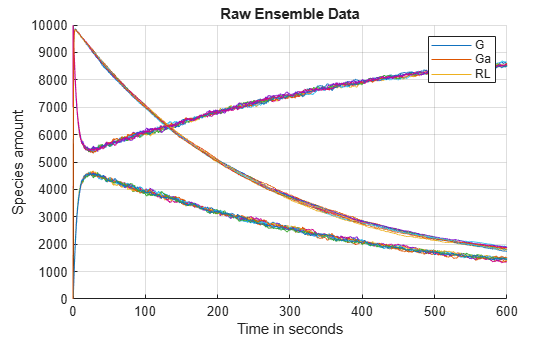### Ensemble Statistics

Let's compute ensemble statistics for these species.

`[timeVals, meanVals, varianceVals] = sbioensemblestats(simdata, speciesNames);`

Let's plot the mean and standard deviation as a function of time.

```figure; plot(timeVals, meanVals); grid on; xlabel('Time in seconds'); ylabel('Mean'); title('Variation of Mean'); legend(speciesNames, 'Location', 'NorthEastOutside');``````figure; plot(timeVals, sqrt(varianceVals)); grid on; xlabel('Time in seconds'); ylabel('Standard Deviation'); title('Variation of Standard Deviation'); legend(speciesNames, 'Location', 'NorthEastOutside');```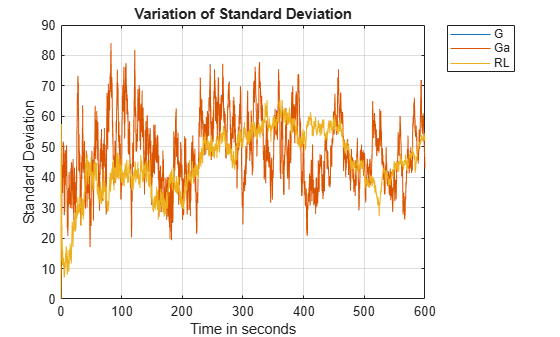From these plots it appears that G and Ga have exactly identical standard deviations, though the variation of their means is different. Let's see if we can find out why that is so. The first step would be to figure out if there is any relationship between them. Let's look at the reactions in the model once again to see if anything is obvious.

`m1.Reactions`
```ans = SimBiology Reaction Array Index: Reaction: 1 L + R <-> RL 2 R <-> null 3 RL -> null 4 Gd + Gbg -> G 5 G + RL -> Ga + Gbg + RL 6 Ga -> Gd ```

### Moiety Conservation

From the reactions, the relationship between G and Ga is not quite clear. To analyze further we are going to use `sbioconsmoiety` to see if there is any moiety conservation.

`sbioconsmoiety(m1, 'semipos', 'p')`
```ans = 2x1 cell {'G + Gbg' } {'G + Gd + Ga'} ```

From this we can see that 'G + Gd + Ga' is always conserved. But that does not completely explain why the variances of G and Ga are identical. What about Gd? Why doesn't its variance affect G or Ga? To look into it further, let's compute the ensemble statistics for Gd and plot their variation.

```[timeValsGd, meanValsGd, varianceValsGd] = sbioensemblestats(simdata, 'Gd'); figure; plot(timeValsGd, meanValsGd, '-', ... timeValsGd, sqrt(varianceValsGd), 'r:'); axis([-50 600 -500 3000]); xlabel('Time in seconds'); title('Mean and Standard Deviation of Gd'); legend('Mean', 'Standard Deviation')```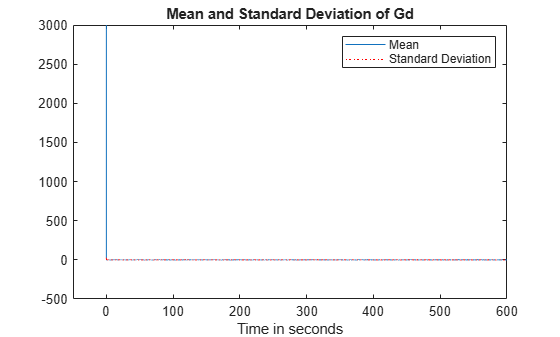### Explanation of Identical Variances

From the plot, it can be seen that Gd starts out with a non-zero value at time = 0, but both its mean and variance approach zero very sharply and stay there. Thus, when Gd stays near zero, the moiety conservation equation reduces to

`$G+Ga\approx constant$`

This means as G goes up Ga goes down by an equal amount and vice versa. This explains why the variances of G and Ga are almost identical. If you look at the data in matrix varianceVals, you'll see that the two variances are very close but not exactly equal. This is due to the presence of Gd which is very close to zero but not exactly zero.

### Ensemble Plots: 2D Distribution Plots

One way to visualize stochastic ensemble data is to plot histograms of species concentrations at particular time points. Each histogram shows the distribution of concentrations for a particular species over the entire ensemble of runs. These histograms may be generated using the SimBiology command `sbioensembleplot`.

Let's create histograms for the species G, Ga, and RL at time t = 10. Note that in this example, we generated the ensemble data without specifying an interpolation option for `sbioensemblerun`. The time vectors for each run within the ensemble are therefore different from each other. `sbioensembleplot` interpolates the simulation data to find species amounts for every run at the precise time t = 10.

`sbioensembleplot(simdata, speciesNames, 10);`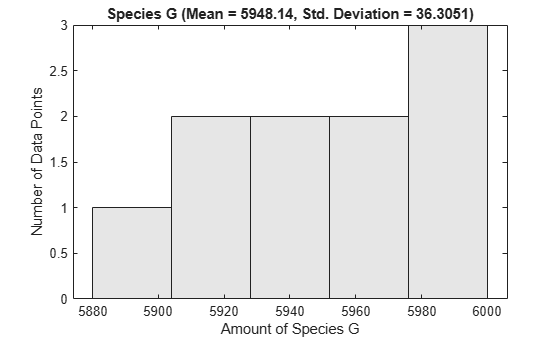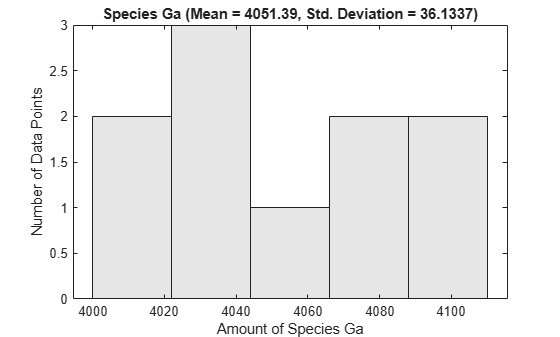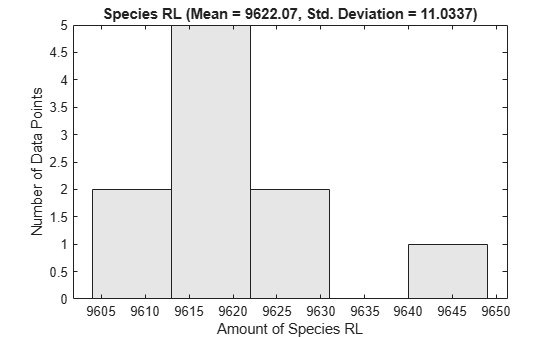### Ensemble Plots: 3D Mountain Plots

It is clear that to get a full understanding of how the distribution changes with time, you will need to make these distribution plots at every time interval of interest. Moreover, the distribution plots need to be seen along with the mean and variance plots. It would be nice to put all of this information together in just one plot.

Well, the 3D ensemble data plots do exactly that. With these 3D plots you can view how mean and variance change as a function of time. In addition, instead of having to plot the distribution of species at every possible time step, in one view you can see how a fitted normal distribution, with the same mean and variance as the actual data, changes with time. The 3D ensemble plot is excellent for getting an overview of how mean, variance and distribution vary as a function of time.

Let's see a 3D ensemble plot of species Ga.

`sbioensembleplot(simdata, 'Ga');`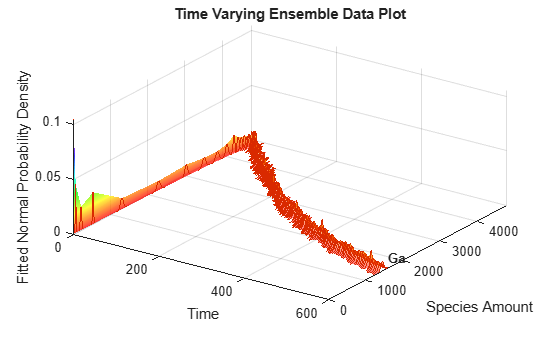This example showed how stochastic ensemble data of SimBiology models can be analyzed using various tools in SimBiology.

##### SupportGet trial now